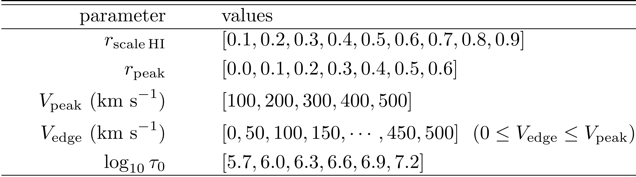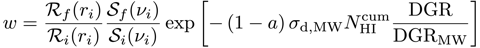LaRT models - Introduction

We provide outputs of our Ly𝛼 radiative transfer calculations for a galaxy toy model, which we perform for reproducing Ly𝛼 properties (spectrum and surface brightness profile) of eight star-forming galaxies at high redshifts (i.e., z=3—6) observed with MUSE (e.g., Leclercq et al. 2017). The paper of this work is under review now (Song, Seon, & Hwang, submitted to ApJ). These outputs can be applied to a larger sample of future observations and can be used for further investigations, for examples, on how galaxies will look like in Ly𝛼 depending on each galaxy model parameter.

We model a galaxy as a halo in which density distributions of Ly𝛼 sources and neutral hydrogen plus dust medium are described with exponential functions. We also consider an outflow of the medium that represents a momentum-driven wind in a gravitational potential well (but simplified as a broken linear function). Our galaxy model is described by five simulation parameters including the scale radius of medium density distribution (rscale,HI), the peak velocity of the velocity profile of medium (Vpeak), the galactic-centric radial distance where the medium velocity reaches its maximum (rpeak), the velocity at the edge (Vedge), and the medium optical depth at the line center (𝜏0). One can implement the effects of Ly𝛼 source distribution (rscale,UV), Ly𝛼 intrinsic frequency distribution, dust (dust-to-gas ratio; DGR), and redshift (z)  through post-processing. Therefore, one can play with nine parameters (five simulation parameters and four post-processing parameters). It should be noted that the medium temperature is fixed at 104K.

We run a Monte Carlo radiative transfer code for Ly𝛼, LaRT, developed by Kwang-il Seon (Seon & Kim, to be submitted), with this galaxy model for a given simulation parameter set. In the code, the regular hexagon that circumscribes the model galaxy is discretized into 1283 cells. The galaxy model parameter grid is summarized in the following table. In total, we run 13,230 simulations with 106 photons per simulation. An output contains the initial 3D radial position (ri), initial frequency (xfreqi), final frequency (xfreqf), cumulative hydrogen column density (cumNHI), and final projected 2D radial position (rf). It should be noted that the  ri  and  rf  are normalized to the size of the galaxy (rmax = 1).

The effects of the four post-processing parameters can be implement by adjusting the weight of each photon, as described in Song, Seon & Hwang.

Here, R and S are the intrinsic spatial and spectral distributions of Ly𝛼 sources, respectively. Here, the subscripts i and f indicate the initial (that is chosen as an input for the simulation run) and final choices, respectively. DGR/DGRMW is the amount of dust relative to gas normalized by the value of the Milky Way, and 𝜎d,MW is the dust cross section per neutral hydrogen of the Milky Way. In our simulation, Ri and Si are uniform distribution functions of r and x (dimensionless frequency = (𝜈 - 𝜈𝛼)/𝛥𝜈D) respectively, within given ranges (0 < r/rmax < 1,  -30 < x <30). This is convenient to adopt other distributions through post-processing. The exponential term can be simply rewritten as exp((1 a)𝜏d), which depicts how much fraction of each photon would be survived after its travel in the galaxy. In the post-processing, we assume that dust scatters photons to the direction of its original propagation (perfect forward scattering). At far ultraviolet wavelengths, scattering is indeed strongly forward-directed (Seon & Draine 2016). The dust extinction cross-section and albedo of the Milky Way are 𝜎d,MW = 1.61×1021 cm2/H and aMW = 0.325, respectively (Weingartner & Draine 2001; Draine 2003).